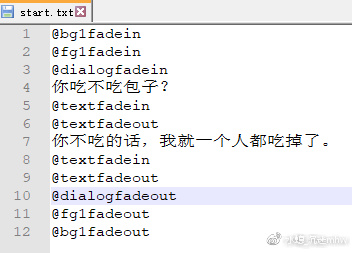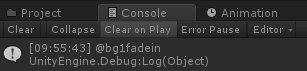04简单的脚本控制//设定一个放脚本的容器

string[] myscript;

//设定脚本读到的行数

int scriptnum = 0;

//从txt里读取脚本然后分行放入myscript里

{

//读取start.txt

//分行放入容器

myscript = Regex.Split(t1.text, "\r\n", RegexOptions.IgnoreCase);

//将脚本执行行数放在最开始

scriptnum = 0;

}

(类型)

Regex是System里的一个类，想要直接用它需要在文件头加上using System.Text.RegularExpressions;不然就必须要写全名字System.Text.RegularExpressions.Regex这样才能用……

Split是Regex用于分割字符串的一个静态函数，它会把一个长字符串分割成字符串数组(string[])返回回来

…………以此类推

class a1

{

int v1=1;

void b1()

{

v1=v1+1;

int v2=2;

}

}

v1被定义在a1的{}里边，也就是说在这花括号里边的所有位置，v1都是有效存在的，你什么时候都可以直接用它，包括在b1这个function里边也可以直接用它

//开始执行脚本

void startscript()

{

while(onscript())

{

}

}

//设定一个存放对话框要放什么文字的变量

string dtext = "";

bool onscript()

{

if(scriptnum>=myscript.Length)

{

//当执行行数超过脚本总行数的时候返回false停止继续执行

return false;

}

//将当前要执行的那一行的脚本赋值给叫做line的变量

string line = myscript[scriptnum];

//因为已经读取了当前要执行的那行，所以执行行数增加1，这样下次就会读取下一行了。scriptnum++相当于scriptnum=scriptnum+1

scriptnum++;

//将这行脚本在console里输出出来

Debut.Log(line);

if(line.Length==0)

{

//如果这行为空就跳过这行

return true;

}

else if(line.Substring(0,1)=="@")

{

//如果行首有@就作为一个function执行

SendMessage(line.Substring(1));

return false;

}

else

{

//如果行首没有@就把文字赋值给dtext等待对话框里显示

dtext = line;

return true;

}

}onscript里边我们用到了if结构，因为太基础了我不是很想啰嗦了……请看c#语法https://docs.microsoft.com/zh-cn/dotnet/csharp/tutorials/intro-to-csharp/branches-and-loops?tutorial-step=1

​//设定一个判断是否在等待点击的变量

bool onwaitclick = false;

{

GameObject temp = GameObject.Find("ui_dialog_text");

Animator an = temp.GetComponent<Animator>();

an.SetInteger("a_num", 1);

//将dtext里边存的文字赋值给画面,为了能够使用Text，需要在文件头加上using UnityEngine.UI;

temp.GetComponent<Text>().text = dtext;

//设定开始等待点击

onwaitclick = true;

}

void Update () {

//当正在等待点击的时候鼠标点击了就继续读下一行脚本

if (onwaitclick && Input.GetMouseButton(0))

{

onwaitclick = false;

startscript();

}

}

Input是unity里管理输入事件的类，具体见https://docs.unity3d.com/ScriptReference/Input.html

IEnumerator waittime(float time)

{

yield return new WaitForSeconds(time);

startscript();

}

IEnumerator这个类型的函数里，我们可以用yiele return来设定等待时间。

void b1()

{

int a=1;

int b=2;

}

IEnumerator b1()

{

int a=1;

yield return new WaitForSeconds(1f);

int b=2;

}

using System.Collections;

using System.Collections.Generic;

using UnityEngine;

using System.Text.RegularExpressions;

using UnityEngine.UI;

public class root_script : MonoBehaviour {

// Use this for initialization

void Start ()

{

startscript();

}

// Update is called once per frame

void Update ()

{

//当正在等待点击的时候鼠标点击了就继续读下一行脚本

if (onwaitclick && Input.GetMouseButton(0))

{

onwaitclick = false;

startscript();

}

}

{

GameObject temp = GameObject.Find("bg1");

Animator an = temp.GetComponent<Animator>();

an.SetInteger("a_num", 1);

StartCoroutine(waittime(1f));

}

{

GameObject temp = GameObject.Find("bg1");

Animator an = temp.GetComponent<Animator>();

an.SetInteger("a_num", 2);

StartCoroutine(waittime(1f));

}

{

GameObject temp = GameObject.Find("fg1");

Animator an = temp.GetComponent<Animator>();

an.SetInteger("a_num", 1);

StartCoroutine(waittime(1f));

}

{

GameObject temp = GameObject.Find("fg1");

Animator an = temp.GetComponent<Animator>();

an.SetInteger("a_num", 2);

StartCoroutine(waittime(1f));

}

{

GameObject temp = GameObject.Find("ui_dialog");

Animator an = temp.GetComponent<Animator>();

an.SetInteger("a_num", 1);

StartCoroutine(waittime(1f));

}

{

GameObject temp = GameObject.Find("ui_dialog");

Animator an = temp.GetComponent<Animator>();

an.SetInteger("a_num", 2);

StartCoroutine(waittime(1f));

}

bool onwaitclick = false;

{

GameObject temp = GameObject.Find("ui_dialog_text");

temp.GetComponent<Text>().text = dtext;

Animator an = temp.GetComponent<Animator>();

an.SetInteger("a_num", 1);

onwaitclick = true;

}

{

GameObject temp = GameObject.Find("ui_dialog_text");

Animator an = temp.GetComponent<Animator>();

an.SetInteger("a_num", 2);

StartCoroutine(waittime(0.5f));

}

//设定一个放脚本的容器

string[] myscript;

//设定脚本读到的行数

int scriptnum = 0;

//从txt里读取脚本然后分行放入myscript里

{

//读取start.txt

//分行放入容器，为了使用Regex，需要在文件头加上using System.Text.RegularExpressions;

//这里的分隔符如果是mac系统可能要改成"\n"才能正常运行

myscript = Regex.Split(t1.text, "\r\n", RegexOptions.IgnoreCase);

//将脚本执行行数放在最开始

scriptnum = 0;

}

//开始执行脚本

void startscript()

{

while(onscript())

{

}

}

//设定一个存放对话框要放什么文字的变量

string dtext = "";

bool onscript()

{

if(scriptnum>=myscript.Length)

{

return false;

}

string line = myscript[scriptnum];

print(line);

scriptnum++;

if(line.Length==0)

{

//如果这行为空就跳过这行

return true;

}

else if(line.Substring(0,1)=="@")

{

//如果行首有@就作为一个function执行

SendMessage(line.Substring(1));

return false;

}

else

{

//如果行首没有@就把文字赋值给dtext等待对话框里显示

dtext = line;

return true;

}

}

IEnumerator waittime(float time)

{

yield return new WaitForSeconds(time);

startscript();

}

}bool onscript()

{

if(scriptnum>=myscript.Length)

{

return false;

}

string line = myscript[scriptnum];

scriptnum++;

if(line.Length==0)

{

//如果这行为空就跳过这行

return true;

}

else if(line.Substring(0,1)=="@")

{

//如果行首有@就作为一个function执行

//建立一个叫做elm的字典来放置脚本传进来的参数

Dictionary<string, string> elm = new Dictionary<string, string>();

//先将这一行脚本用空格分割开。

string[] temparr = Regex.Split(line.Substring(1), " ", RegexOptions.IgnoreCase);

//分割出来的第一个字串是function的名字，放到叫做macroname的变量里等会用。

string macroname = temparr;

//如果后边还有参数的话就再用=分割开它们，把它们放进elm里

if (temparr.Length > 1)

{

for (int i = 1; i < temparr.Length; i++)

{

string[] temparr1 = Regex.Split(temparr[i], "=", RegexOptions.IgnoreCase);

elm[temparr1] = temparr1;

}

}

//按照有参数和没参数两种来使用SendMessage

if (elm.Count > 0)

{

SendMessage(macroname,elm);

}

else

{

SendMessage(macroname);

}

return false;

}

else

{

//如果行首没有@就把文字赋值给dtext等待对话框里显示

dtext = line;

return true;

}

}

{

GameObject temp = GameObject.Find("bg1");

//读取通过elm传进来的图片名的图片，把它挂在bg1上显示出来

Animator an = temp.GetComponent<Animator>();

an.SetInteger("a_num", 1);

StartCoroutine(waittime(1f));

}​之后哪怕再有bg3 bg4 bg5都可以随意使用了。

aa